# GP - 8 items

Determine the first eight members of a geometric progression if a9=512, q=2

Correct result:

a1 =  2
a2 =  4
a3 =  8
a4 =  16
a5 =  32
a6 =  64
a7 =  128
a8 =  256

#### Solution:

${a}_{1}=512\mathrm{/}{2}^{9-1}=2$
${a}_{2}=512\mathrm{/}{2}^{9-2}=4$
${a}_{3}=512\mathrm{/}{2}^{9-3}=8$
${a}_{4}=512\mathrm{/}{2}^{9-4}=16$
${a}_{5}=512\mathrm{/}{2}^{9-5}=32$
${a}_{6}=512\mathrm{/}{2}^{9-6}=64$
${a}_{7}=512\mathrm{/}{2}^{9-7}=128$
${a}_{8}=512\mathrm{/}{2}^{9-8}=256$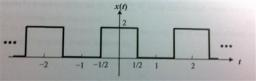We would be pleased if you find an error in the word problem, spelling mistakes, or inaccuracies and send it to us. Thank you!## Next similar math problems:

• Five membersWrite first 5 members geometric sequence and determine whether it is increasing or decreasing: a1 = 3 q = -2
• Gp - 80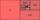Sum of the first four members of a geometric progression is 80. Determine they if we know that the fourth member is nine times greater than the second.
• Geometric sequenceIn the geometric sequence is a4 = 20 a9 = -160. Calculate the first member a1 and quotient q.
• Five element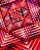The geometric sequence is given by quotient q = 1/2 and the sum of the first six members S6 = 63. Find the fifth element a5.
• Geometric progressionIn geometric progression, a1 = 7, q = 5. Find the condition for n to sum first n members is: sn≤217.
• Three members GPThe sum of three numbers in GP (geometric progression) is 21 and the sum of their squares is 189. Find the numbers.
• Geometric sequence 3In geometric sequence is a8 = 312500; a11= 39062500; sn=1953124. Calculate the first item a1, quotient q and n - number of members by their sum s_n.
• Sum of GP members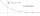Determine the sum of the GP 30, 6, 1.2, to 5 terms. What is the sum of all terms (to infinity)?
• Tenth memberCalculate the tenth member of geometric sequence when given: a1=1/2 and q=2
• GP - three membersThe second and third of a geometric progression are 24 and 12(c+1) respectively, given that the sum of the first three terms of progression is 76 determine value of c
• Q of GP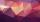Calculate quotient of geometric progression if a1=5 a a1+a2=12.
• Geometric sequence 4It is given geometric sequence a3 = 7 and a12 = 3. Calculate s23 (= sum of the first 23 members of the sequence).
• Geometric sequence 5About members of geometric sequence we know: ? ? Calculate a1 (first member) and q (common ratio or q-coefficient)
• GP membersThe geometric sequence has 10 members. The last two members are 2 and -1. Which member is -1/16?
• If the 3If the 6th term of a GP is 4 and the 10th is 4/81, find common ratio r.
• Eight palm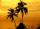There grows 8 palms by the sea. At the first sitting one parrot, on second two, on third sits four parrots on each other twice the previous parrots sitting on a previous palm. How many parrots sitting on eighth palm?
• Find next memberFind x if the numbers from a GP 7, 49, x.